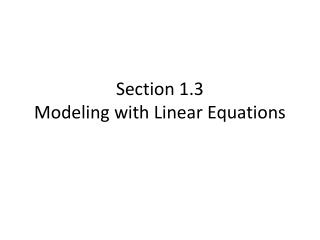DownloadDownload PresentationSection 1.3 Modeling with Linear Equations

# Section 1.3 Modeling with Linear Equations

Télécharger la présentation## Section 1.3 Modeling with Linear Equations

- - - - - - - - - - - - - - - - - - - - - - - - - - - E N D - - - - - - - - - - - - - - - - - - - - - - - - - - -
##### Presentation Transcript

1. Section 1.3Modeling with Linear Equations

2. THE RULES A problem will appear on the screen. Use discussion in your group and paper to solve the problem. You have three minutes maximum to solve the problem. When you have your answer, write it down on the white board and hold it up so the teacher can see. First group to get a correct answer gets three points. Second group to get a correct answer gets two points. Any other group who gets a correct answer gets one point. ONLY ONE ANSWER MAY BE SUBMITTED PER QUESTION. The group with the most points at 9:45 gets 5 points extra credit per person, second place 4 points per person, etc.

3. Question 1 The sum of three consecutive numbers is 804. Find the numbers.

4. Question 2 70 is 40% of what number?

6. Question 4 A picture frame has a total perimeter of 48.6 inches. The height of the frame is 0.62 times its width. Find the height (h) and the width (w) of the frame. Note, your answer should look like h = ___ and w = ___

7. Question 5 Two cars start at a given point and travel in the same direction at average speeds of 40 mph and 55 mph. How many MINUTES elapse before the two cars are five miles apart?

8. Question 6* A LWE bus driver traveled at an average speed of 55 mph on a 200 miles trip to U of I to pick up students. Since I-57 was closed on the way back, he could only average 40 mph on the return trip. Find the average speed for the round trip. Round your answer to the nearest whole number.

9. Question 7 To obtain the height of a tree, you measure the tree’s shadow and find that it is 8 feet long. You also measure the shadow of a 2 foot lamp post and find that it is 9 INCHES long. How many feet tall is the tree?

10. Question 8 An apple store has \$20,000 of inventory in ipods and iphones. The profit on an ipod is 25% and the profit on an iphone is 17%. The profit for the entire inventory is 20%. How much money was originally invested in each? Your answer should read Ipods - \$_________ Iphones \$___________

11. Question 9 Simplify the expression below

12. Question 10* A rectangular kiddie pool is 12 feet long, 3 feet deep, and 3 feet wide. If 1 gallon = 0.13368 cubic foot, find the depth of the water when the pool contains 70 gallons of water. Round your answer to TWO DECIMAL PLACES.

13. Question 11 Solve the equation below for b, writing your answer as a single simplifed fraction:

14. Question 12 Solve the equation below for r, writing your answer as a single simplifed fraction: